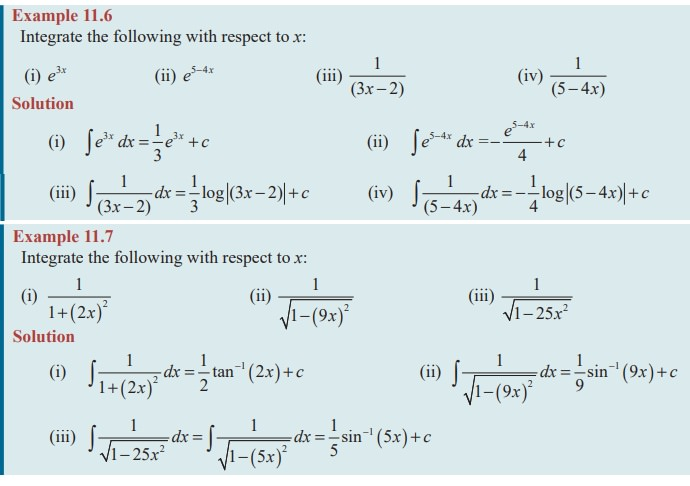Home | | Maths 11th std | Integrals of the Form (ax + b)

# Integrals of the Form (ax + b)

Integrals of the Form ‚ąę f ( ax + b )dx

Integrals of the Form ‚ąę f ( ax + b )dx

We know thatIt is clear that whenever a constant is added or subtracted with the independent variable x, the fundamental formulae remain the same.Here, if any constant is multiplied with the independent variable x, then the same fundamental formula can be used after dividing it by the coefficient of xThe above formula can also be derived by using substitution method, which will be studied later.Example 11.5

Integrate the following with respect to x:

(i) sin(2x + 4) (ii) sec2 (3 + 4x) (iii) cosec( ax + b ) cot( ax + b)

SolutionEXERCISE 11.2

Integrate the following functions with respect to x:Tags : Equation, Solved Example Problems | Mathematics , 11th Mathematics : UNIT 11 : Integral Calculus
Study Material, Lecturing Notes, Assignment, Reference, Wiki description explanation, brief detail
11th Mathematics : UNIT 11 : Integral Calculus : Integrals of the Form (ax + b) | Equation, Solved Example Problems | Mathematics